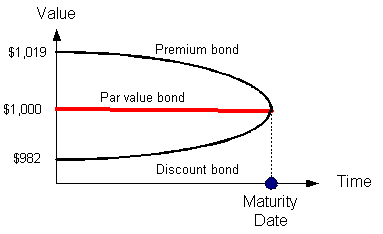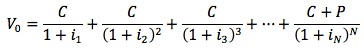### Why should I choose AnalystNotes?

AnalystNotes specializes in helping candidates pass. Period.

##### Subject 2. Relationships between Bond Price and Bond Characteristics
Price / Discount Rate Relationship

The value of a bond is equal to the present value of its coupon payments plus the present value of the maturity value.The higher the discount rate, the lower a cash flow's present value and therefore since the value of a security is the present value of the cash flows, the higher the discount rate, the lower a security's value.

Example

A 1-year, semi-annual-pay bond has a \$1,000 face value and a 10% coupon.

• At a discount rate of 8%, the bond value is \$1,019 (premium).
• At a discount rate of 10%, the bond value is \$1,000 (par).
• At a discount rate of 12%, the bond value is \$982 (discount).

The degree of price change is not always the same for a particular bond. The price/yield relationship for an option-free bond is convex. In other words, this is not a straight-line relationship.For a given change in yield, the price increases by more than it decreases. P1 - P > P - P2.

Option-free bonds exhibit positive convexity, which means that for a large change in interest rates, the amount of price appreciation is greater than the amount of price depreciation. This also means that the price change is greater when the level of required yield is low (and vice versa).

Coupon and Maturity Effects

All else being equal,

• Maturity effect: The longer the term to maturity, the greater the price volatility.
• Coupon effect: The lower the coupon rate, the greater the price volatility.

Constant-Yield Price Trajectory

As a bond moves closer to its maturity date, its value changes. More specifically, assuming that the discount rate does not change, a bond's value:

• decreases over time if the bond is selling at a premium
• increases over time if the bond is selling at a discount
• is unchanged if the bond is selling at par value

At the maturity date, the bond's value is equal to its par value ("pull to par value").Pricing Bonds with Spot Rates

The valuation approach illustrated so far is the traditional approach, which uses a single interest rate to discount all of a bond's cash flows. It views all cash flows of a bond as the same, regardless of their timing. In reality, however, each individual cash flow of the bond is unique. Therefore, using a single discount rate in the bond valuation model may result in a mis-priced bond, thereby creating arbitrage opportunities.

The arbitrage-free approach values a bond as a package of cash flows, with each cash flow viewed as a zero-coupon bond and discounted at its own unique discount rate. These spot rates are used to discount cash flows to get the arbitrage-free value of a bond.

The arbitrage-free approach has three steps.

• Take each individual cash flow of a coupon as a stand-alone zero-coupon bond. Each cash flow is the face value of the corresponding zero.
• Value each zero-coupon bond by discounting its cash flow at the corresponding spot rate.
• Add up the value of each zero to calculate the total value of the zero-coupon bond portfolio.Learning Outcome Statements

identify the relationships among a bond's price, coupon rate, maturity, and market discount rate (yield-to-maturity);

define spot rates and calculate the price of a bond using spot rates;

CFA® 2023 Level I Curriculum, Volume 4, Module 44

User Comment
Bobokoko So, if you hold a bond til maturity and you have this convergence, what happens to interest rate risk??? If a bond is guaranteed to pay par, what happened to the risk of higher rates??

Let's suppose 5Yr semi-annual bond has been selling at par for past 4.5Yrs (N=9) becaus coupons rate and market rate both were at 10%. Now when only 6 more months are left i.e. N=1, market rate jumps to 15%, i.e. I/Y=7.5%

Let calculate the price of the bond using above information.

FV = 1000
I/Y = 7.5%
N = 1
PMT= 50
PV = 976.74

Do you see the PV is very close to the FV.

Now try the N=0, you'll get PV=FV.

Hence, market rate risk needs times to work its effects on the bond price. If the market rate was even at 20%, but there was no time left, i.e N, then it can't impact much on the price of the bond.
Seemorr I see what you're asking ... if the bond is trading at par then the market has determined the coupon payments represent appropriate compensation (i.e., the bond yield is the correct discount rate).
hit81 Both Premium bonds( Discount bonds) tends to decrease(increase) and slightly adjust to the price somewhere near Parvalue of the bond , when the respective bond approaching Maturity date, is this correct?
johntan1979 My advice is for you guys to go back a few chapters and get your basics right. If at this point you still have all these questions means you are not ready to proceed further.
jonan203 i agree with johnny, you guys (with the entry level questions) need to reread the chapter on quantitative methods...
guest I'm hoping we're gonna learn Option Free Bond soon. I'm not exactly sure what it means.
JKS014777 hey guys, Is anybody familiar with how to get the explanation of the question answer from the list of basic question ?
sshetty2 There is no interest rate risk on a bond held to maturity
sshetty2 On a zero coupon bond held to maturity*